Intro to Regrouping Video for Kids | K, 1st & 2nd Grade
1%
It was processed successfully!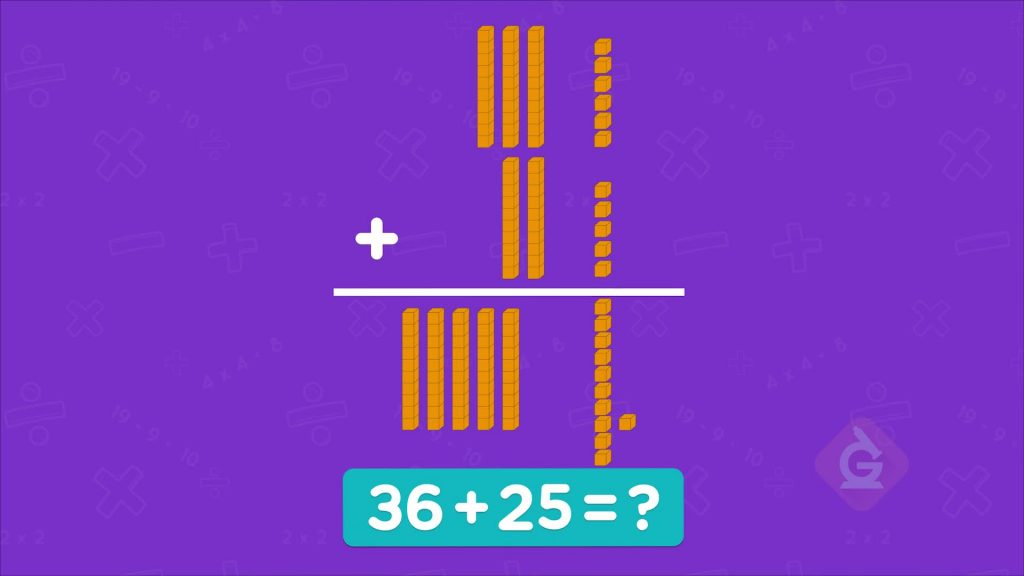WHAT IS INTRO TO REGROUPING (WHEN ADDING & SUBTRACTING)?

You have learned before that 10 ones is equal to 1 ten. When you add, you can regroup 1 ten as 10 ones. When you subtract, you can regroup 10 ones as 1 ten.

To better understand Regrouping when Adding & Subtracting…

WHAT IS INTRO TO REGROUPING (WHEN ADDING & SUBTRACTING)?. You have learned before that 10 ones is equal to 1 ten. When you add, you can regroup 1 ten as 10 ones. When you subtract, you can regroup 10 ones as 1 ten. To better understand Regrouping when Adding & Subtracting…

## LET’S BREAK IT DOWN!

### Place value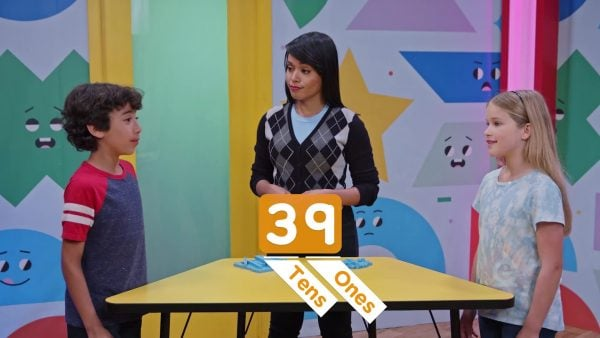Place value tells us the value of each digit based on its position in a number. For example, 39 has 3 in the tens place and 9 in the ones place.

Place value Place value tells us the value of each digit based on its position in a number. For example, 39 has 3 in the tens place and 9 in the ones place.

### What is regrouping?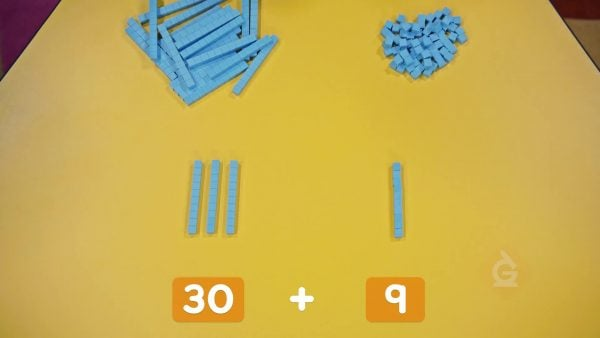When we have more than 10 ones, we can regroup some of them to make a ten. For example, if we have 13 ones, we can regroup them as 1 ten and 3 ones.

What is regrouping? When we have more than 10 ones, we can regroup some of them to make a ten. For example, if we have 13 ones, we can regroup them as 1 ten and 3 ones.

### Regroup 53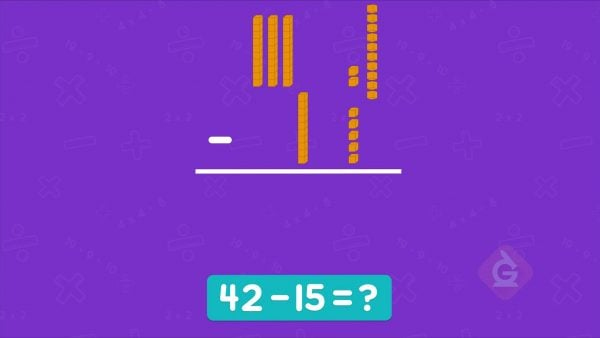53 is 5 tens and 3 ones. We can regroup 1 ten as 10 ones. 53 is 4 tens and 13 ones. 53 is also 53 ones. We can regroup 10 ones as 1 ten. 53 is 1 ten and 43 ones.

Regroup 53 53 is 5 tens and 3 ones. We can regroup 1 ten as 10 ones. 53 is 4 tens and 13 ones. 53 is also 53 ones. We can regroup 10 ones as 1 ten. 53 is 1 ten and 43 ones.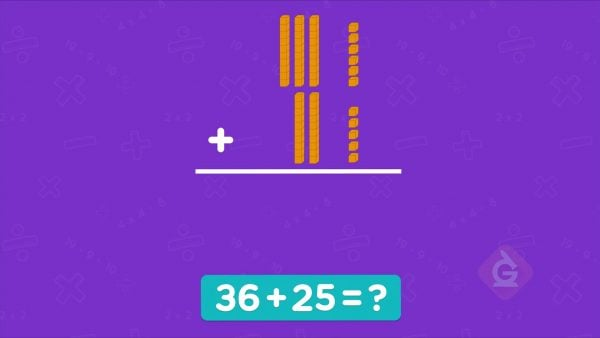Add 36 + 25. Add the ones: 6 ones + 5 ones = 11 ones. Regroup 11 ones to make 1 ten and 1 one. Add the tens: 3 + 2 = 5 tens. 5 tens + 1 ten + 1 one is 6 tens and 1 one, or 61. 36 + 25 = 61.

Regroup when adding Add 36 + 25. Add the ones: 6 ones + 5 ones = 11 ones. Regroup 11 ones to make 1 ten and 1 one. Add the tens: 3 + 2 = 5 tens. 5 tens + 1 ten + 1 one is 6 tens and 1 one, or 61. 36 + 25 = 61.

### Regroup when subtracting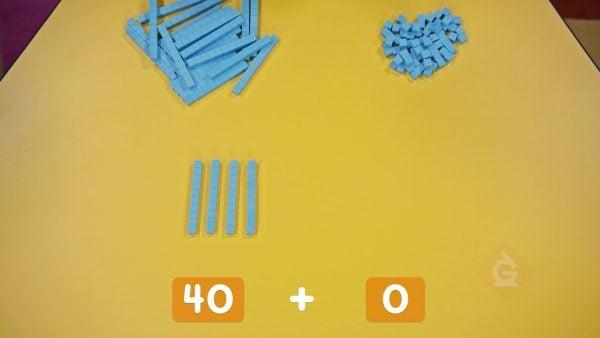Subtract 42 – 15. Try to subtract the ones. We can't take away 5 ones from 2 ones! Regroup 1 ten in 42 into 10 ones. Now we have 12 ones. 12 – 5 = 7 ones. Subtract the remaining tens. 3 tens – 1 ten = 2 tens. There are 2 tens and 7 ones left, or 27. 42 – 15 = 27.

Regroup when subtracting Subtract 42 – 15. Try to subtract the ones. We can't take away 5 ones from 2 ones! Regroup 1 ten in 42 into 10 ones. Now we have 12 ones. 12 – 5 = 7 ones. Subtract the remaining tens. 3 tens – 1 ten = 2 tens. There are 2 tens and 7 ones left, or 27. 42 – 15 = 27.

## INTRO TO REGROUPING (WHEN ADDING & SUBTRACTING) VOCABULARY

Subtract
Find the difference between two amounts.
Put together two amounts.
Place value
The value of each digit based on its position in a number.
Ones place
Has a value of one.
Tens place
Has a value of ten ones.
Regrouping
Representing tens using ones, or representing ones using tens.
The result of subtracting.

## INTRO TO REGROUPING (WHEN ADDING & SUBTRACTING) DISCUSSION QUESTIONS

### Do you need to regroup when you add 25 + 15?

Yes! After adding, I have 3 tens blocks and 10 ones blocks. 10 ones blocks can be regrouped into 1 tens block, so I have 4 tens blocks, or 40.

### Do you need to regroup to subtract 47 – 12?

No. 7 is greater than 2, so to subtract the ones, I can subtract 7 – 2. 4 is also greater than 1 so to subtract the tens, I can subtract 4 – 1. The answer is 35.

### Do you need to regroup to subtract 47 – 19?

Yes. 7 is less than 9, so I cannot subtract 7 – 9. I have to borrow a ten and regroup it as 10 ones. I take one of the 4 tens blocks and regroup it into 10 ones blocks. Now I have 3 tens blocks and 17 ones block. I need to subtract 1 ten and 9 ones. To subtract the ones, I can subtract 17 – 9. To subtract the tens, I can subtract 3 – 1. The answer is 28.

### Cathy wanted to add 28 + 43, so she regrouped everything into ones blocks and counted 71 blocks in all. She then regrouped them again into 7 tens blocks and 1 ones block. Did she get the right answer? Did she do it right?

Cathy got the right answer, but she did not have to regroup the two numbers into ones blocks first. She could have added together the tens and the ones, and then regrouped 10 of the ones into 1 ten.

### Joey wanted to subtract 34 – 27, so he took 27 and regrouped one of the tens into 10 ones. What did he do wrong?

It does not help to regroup parts of 27. He should regroup 1 ten from 34 into 10 ones, because he needs more than 4 ones.
X

## Success

We’ve sent you an email with instructions how to reset your password.
Ok